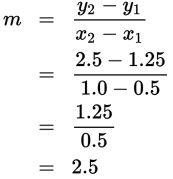# SAT Math Multiple Choice Question 326: Answer and Explanation

### Test Information

Question: 326

11. Acetaminophen is one of the most common drugs given to children and one of the most difficult to give correctly because it's sold in several different forms and different concentrations. For example, the old concentration given by dropper was 90 mg of acetaminophen per 1 ml of liquid, while the new concentration given by syringe is 160 mg per 5 ml. Several dosages are shown in the table below.

 Infant Acetaminophen Dosages Age 0–3 mo 4–11 mo 12–23 mo Dropper 0.5 ml 1.0 ml 1.5 ml Syringe 1.25 ml 2.5 ml 3.75 ml

Which linear function represents the relationship between the amount of liquid in the dropper, d, and the amount of liquid in the syringe, s?

• A. s = 0.4d
• B. s = 1.25d
• C. s = 2d
• D. s = 2.5d

Explanation:

D

Difficulty: Medium

Category: Heart of Algebra / Linear Equations

Strategic Advice: Don't let all the contextual information confuse you. The question at the end tells you that you are looking for the linear relationship between the pairs of numbers in the last two rows of the table. This amounts to writing an equation in the form y = mx + b.

Getting to the Answer: Take a peek at the answers-none of the equations have a y-intercept, so all you need to do is write the equation y = mx, or in this case, s = md. To find m, use any two ordered pairs from the table and the slope formula. Be careful-d represents x in the equation, so the dropper amounts should be written first in the ordered pairs. Using (0.5, 1.25) and (1.0, 2.5), the slope is:This means the equation is s = 2.5d.